# Shiny, R and HTML: Merging Data Science and Web Development

Programming

Share:

#### Free JavaScript Book!Write powerful, clean and maintainable JavaScript.

RRP \$11.95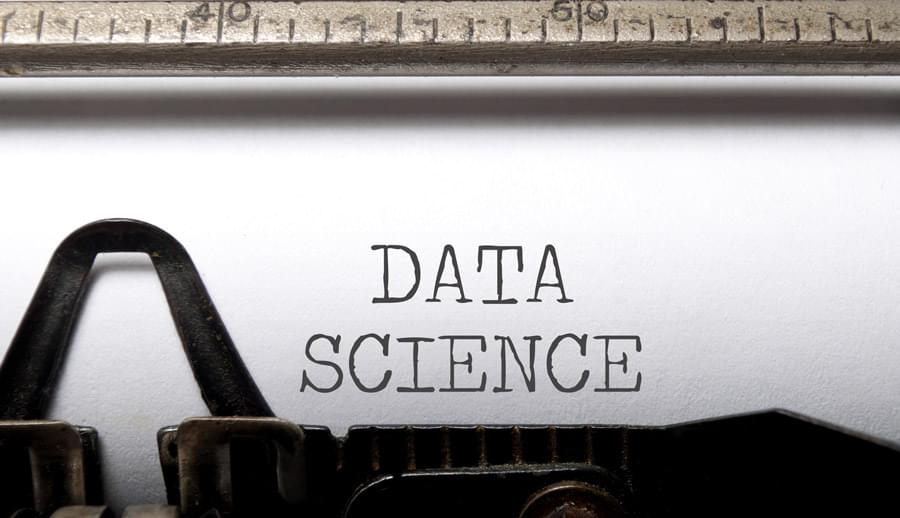With the advent of data science and the increased need to analyze and interpret vast amounts of data, the R language has become ever more popular. However, there’s increasingly a need for a smooth interaction between statistical computing platforms and the web, given both 1) the need for a more interactive user interface in analyzing data, and 2) the increased role of the cloud in running such applications.

Statisticians and web developers have thus seemed an unlikely mix till now, but make no mistake that the interactions between these two groups will continue to increase as the need for web-based platforms becomes ever more popular in the world of data science. In this regard, the interaction of the R and Shiny platforms is quickly becoming a cornerstone of interaction between the world of data and the web.

In this tutorial, we’ll look primarily at the commands used to build an application in Shiny — both on the UI (user interface) side and the server side. While familiarity with the R programming language is invariably helpful in creating a Shiny app, expert knowledge is not necessary, and this example will cover the building of a simple statistical graph in Shiny, along with some basic commands illustrating how to customize the web page through HTML.

To start off, we must first install the RStudio platform in order to create and run a Shiny Web App. RStudio can be downloaded from the RStudio website.

Once we’ve loaded the RStudio platform, we then create our new Shiny web application by selecting the New File option, and then the Shiny Web App… option.Upon doing that, we’re presented with an interface that allows us to name our application (I’ve named it “OurFirstApp”, but feel free to come up with other creative names!), and we then select Multiple File (ui.R/server.R) as our application type: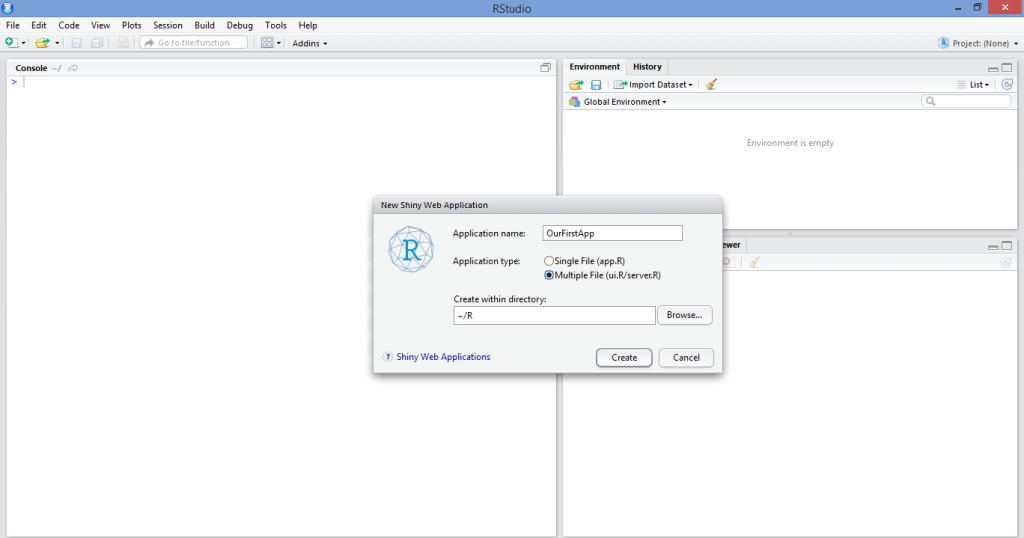While we could select Single File (app.R), this literally just combines the `ui.R` and `server.R` scripts into one. I personally prefer to keep the two separate in order to keep the code less cluttered, but this is a matter of personal preference.

As a side note, there’s also a web platform available on the Shinyapps site, which allows users to share their Shiny applications online, as well as use the rsconnect package to control such applications remotely from the R console. While the methodology for doing this is outside the scope of this article, I definitely intend on expanding this topic further in subsequent articles given interest.

In any case, once we have named our Shiny Web App and opened up the source code, we’re presented with a template app that uses a dataset “Old Faithful Geyser Data” to generate a histogram. For the purposes of this tutorial, we’ll be writing our own app and customizing it according to our needs.

## Running the Application

As already stated, the primary components of a Shiny Web App are the user interface side (`ui.R`) and the server side (`server.R`).

For this particular app, we’re going to create a simple line graph with a plot of `N` observations on the x-axis and probability of occurrence on the y-axis. Essentially, this app creates a slider allowing us to select a value (N) between 1–50. `N` can be thought of as the number of trials, i.e. if a value of 2 is selected, then 2 trials equal a probability of 100 percent. On the other hand, if a value of 50 is selected, then 50 trials must be conducted for the probability of occurrence to equal 100 percent. On a graph where `N = 50`, then sliding back down to `N = 25` would correspond to a 50 percent probability, and the graph updates to reflect this automatically. Note that this is somewhat of an overly simplistic example mathematically, but is merely being used to illustrate how reactivity works in the context of graph generation across the Shiny platform.

While the below code will ultimately be modified slightly to allow for greater customization using HTML, it’s the code used to create both the `ui.R` and `server.R` files:

ui.R

``````#Call the “shiny” library
library(shiny)

#Define the UI for application that draws a probability plot
shinyUI(fluidPage(

#Name the Title of the applicationh
titlePanel("Probability Plots"),

#Create a sidebar with a slider input for number of trials
sidebarLayout(
sidebarPanel(
sliderInput("lambda",
"Number of trials:",
min = 1,
max = 50,
value = 1)
),

#Show a plot of the generated probability plot
mainPanel(
plotOutput("ProbPlot")
)
)
))
``````

server.R

``````#Define the “shiny”, “ggplot2” and “scales” libraries
library(shiny)
library(ggplot2)
library(scales)

#Shiny Application
shinyServer(function(input, output) {

#Create a reactive expression
output\$ProbPlot <- renderPlot({

#Generate lambda value based on input\$lambda from ui.R
n=1:100
lambda <- seq(min(n), max(n), length.out = input\$lambda)
probability=lambda

#Generate the probability plot
plot(probability, type = "o", col = 'blue', border = 'white', xlab="N (Number of Observations)", ylab ="Probability of occurrence")

})

})
``````

We then run our application by selecting the Run External option as below, and then selecting Run App: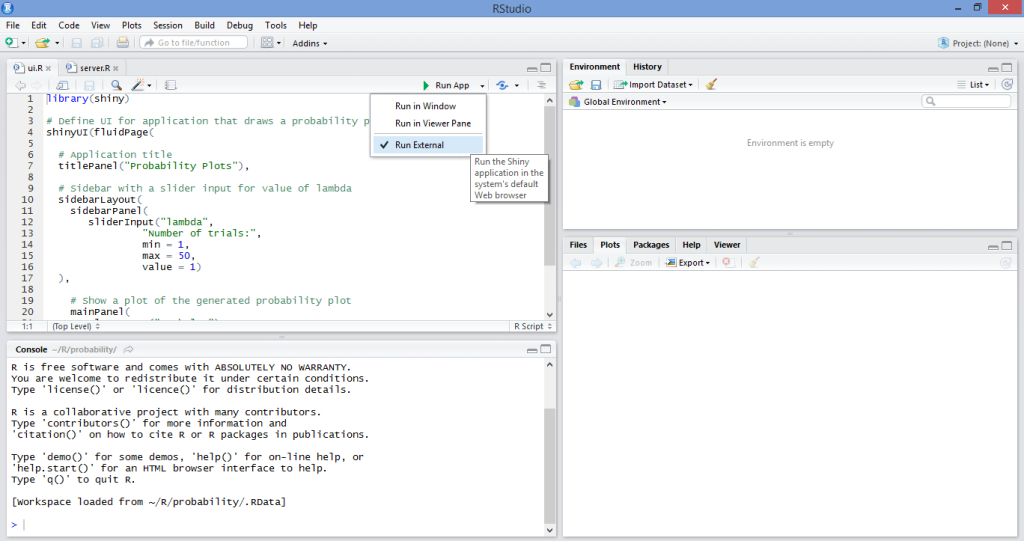Once we run our application, we observe that the graph then updates automatically, allowing us to visually analyze on a line graph the relationship between `N` and probability of occurrence depending on the number of trials we’ve selected: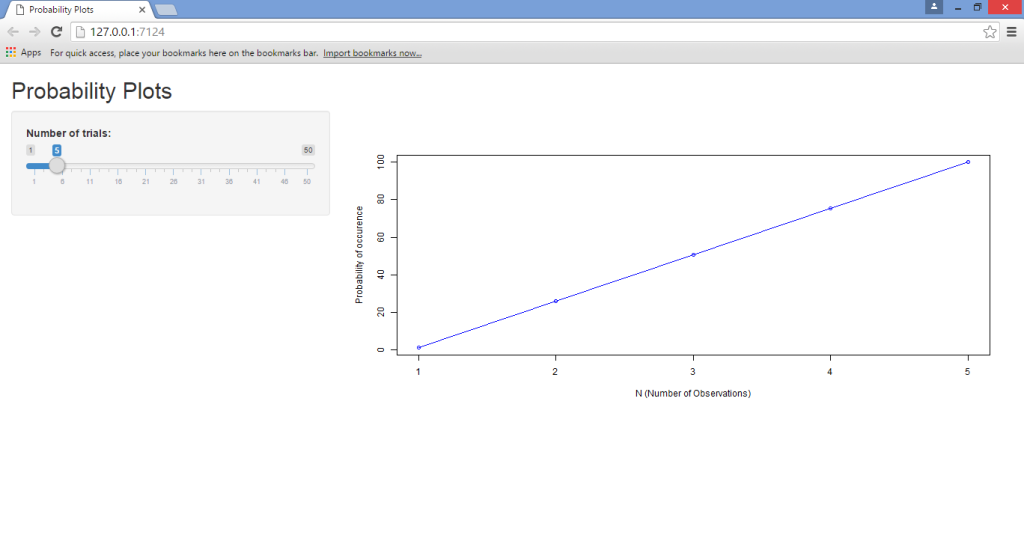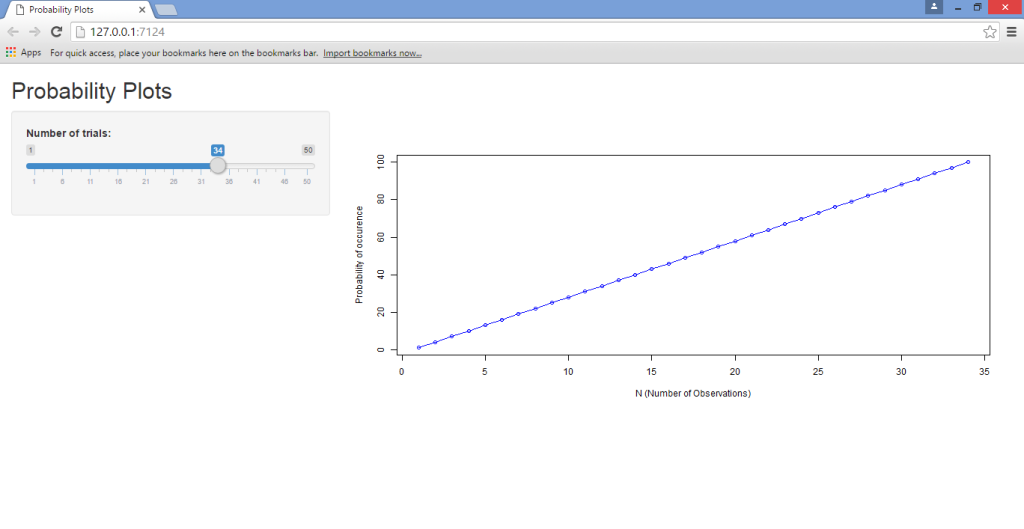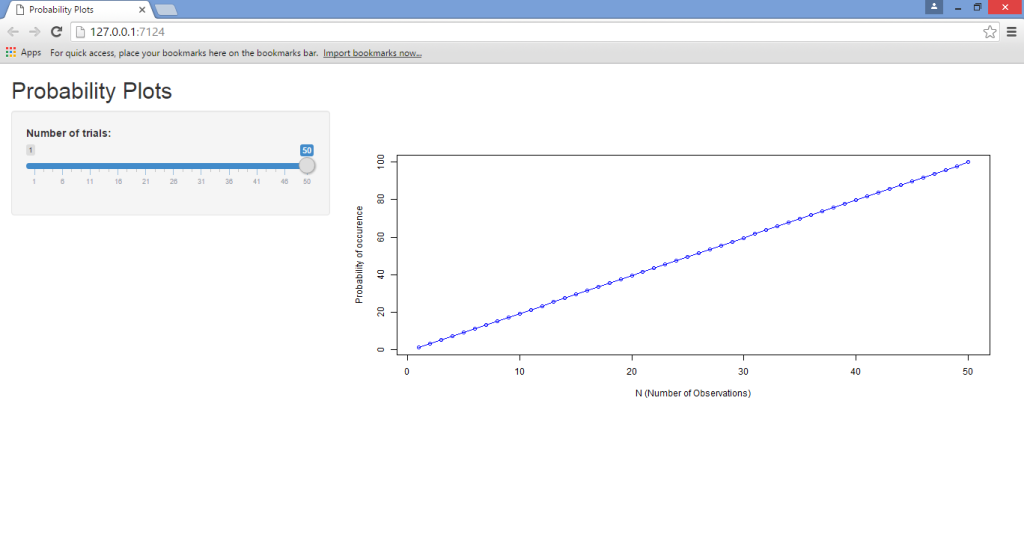As we can see, running this app through Shiny has made it much more user-friendly, in that the slider allows for instantaneous manipulation and observation of the results. This is known as a reactive expression, where the values of one variable automatically update given changes in another. Had we chosen to run this program using R solely, then we would need to type a new value for `N` into the source code each time we wish to run the program, and this is ultimately very monotonous and inefficient — especially for users who don’t already have R language knowledge.

Some points to note from the above scripts:

• The `sidebarLayout` function is what allows us to create the sliding sidebar that enables us to input a specific value for the number of trials. We’re defining this by means of the `min` (minimum value), `max` (maximum value), and `value` (which is the number of values between each value in the series).
• Note that, in the `server.R` script, the code includes both the ggplot2 and scales libraries. When running the program yourself, you will notice that these two libraries are not strictly required to run this particular application. However, I have included them as the ggplot2 library is of great use when running more sophisticated graphs, while the scales library allows for greater customization of graph data that requires different scaling methods across a range of axes or legends.
• Moreover, we see that when we generate a lambda value (for the second-last command of the `server.R` script), we do so by making the variable a function of the minimum and maximum value of `n`, which we have defined as 1 to 100. This is where we define the interaction between `n` and our probability function (known as lambda), which in turn gives rise to a reactive expression:
``````#Generate lambda value based on input\$lambda from ui.R
n=1:100
lambda <- seq(min(n), max(n), length.out = input\$lambda)
probability=lambda
``````

## HTML Customization

As seen above, we created an application that is able to use a reactive expression in updating a line graph and its axes instantaneously. However, given that we are creating a web page with the application after all, suppose that we wish to update the page design using HTML?

The HTML editing that we’ll perform here is, in itself, quite basic. For this tutorial, we’ll simply concentrate on how to edit the titles of the web page in terms of font and color. However, the purpose behind this is to illustrate specifically how to edit the user interface code in R to be able to customize the web page, which is the main takeaway for this tutorial. This involves a slight modification of the `ui.R` file that we generated above to allow for HTML inserts.

One of the most important things to note is that we are only modifying our `ui.R` file — the `server.R` file remains the same as above. This is clearly because HTML is being used for the purposes of modifying the user interface; it’s not being used to modify the parameters of the application itself.

(The template that I’m using to edit the headings of the web page in this instance is based on Chris Beeley’s Second Edition of Web Application Development with R Using Shiny. I have personally found it to be a great guide, and highly recommend it to anyone looking to increase their knowledge of R/Shiny programming and web platforms.)

The modified `ui.R` program that we use to construct the updated web page is as follows:

ui.R

``````#Call the “shiny” library
library(shiny)

# Define UI for application that draws a probability plot
shinyUI(fluidPage(

h2("Probability Plots", style = "font-family: 'Jura'; color: red; font-size: 64px;"),

# Application title
#titlePanel("Probability Plots"),

# Sidebar with a slider input for value of lambda
sidebarLayout(
sidebarPanel(
sliderInput("lambda",
"Number of trials:",
min = 1,
max = 50,
value = 1)
),
# Show a plot of the generated probability plot
mainPanel(
h3("Probability Graph", style = "font-family: 'Jura'; color: blue; font-size: 32px;"),
plotOutput("ProbPlot")
)
)
))
``````

In looking at the difference between this `ui.R` script and that of the previous one, we note that we’re adding to the `shinyUI(fluidPage)` command by defining Google Fonts as the font family using the `tags\$head` command. Moreover, we use the `h2` heading to define the “Probability Plots” heading for the page itself, and subsequently the `h3` heading to define the “Probability Graph” for the graph we have plotted.

Once we run the above code in conjunction with the `server.R` script, we generate the following graph (along with the same slider selection and reactivity as we observed previously):## Conclusion

In this tutorial, we’ve covered:

1. The procedures to install RStudio and load a Shiny Web App in R
2. How to create a plot in Shiny and form reactive expressions
3. Using HTML to customize Shiny webpages.

Needless to say, this application is merely touching the tip of the iceberg in terms of Shiny’s many capabilities with the R platform.

Moreover, Shiny can also be used with other languages such as JavaScript in customizing the user interface of the respective web pages being loaded by the application. However, the above tutorial serves to act as a baseline in developing and running Shiny applications.

I hope this tutorial has been helpful and of interest to you. Let us know what you think in the comments below, and tell us if there are any other Shiny and R topics you’d like us to cover.### Michael Grogan

Michael Grogan is a data science consultant, with a profound passion for statistics and programming. He regularly utilizes programming languages such as Python, R and SQL to analyze a wide range of datasets, and also explores aspects of web development in designing interactive web apps for data science analytics. Find him at michaeljgrogan.com.

#### New books out now!